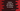# Python find the average of all numbers in a string## Python find the average of all numbers in a string:

This post will show you how to find the average of all numbers in an alphanumeric string in Python. We will write one program that will take one string as input from the user and it will print the average of all numbers found in that string.

For example, if the string is hello123, it will print :2.0. Because, in hello123, we have three digits: 1, 2, and 3. The sum of these digits is 1 + 2 + 3 = 6. So, the average is 6/3 i.e. 2.

### Algorithm to use:

We will use the below algorithm to do this:

• Take one string as input from the user and store that in a variable.
• Initialize two variables sum and count as 0. These variables are to store the sum of all digits and total count of digits.
• Iterate through the characters of the string one by one. For each character, check if it is a diigit or not. If it is a digit, add it to the sum variable. Also, increment the count variable by 1.
• Once the loop ends, print the average value, i.e. divide sum by count.

That’s it.

### Python program:

Below is the complete python program that uses the above algorithm:

``````def find_avg_sum(str):
sum = 0
count = 0
for ch in str:
if ch.isdigit():
sum += int(ch)
count += 1
return sum/count

given_str = input('Enter a string: ')

print(find_avg_sum(given_str))``````

Here,

• It asks the user to enter a string and stores it in given_str variable once the user enters it.
• This string is passed to the method findavgsum. This method is used to find the average of all numbers found in the provided string.

• sum and count variables are to store the sum of all numbers found in the string and the total count of all numbers.
• The for in loop is used to iterate through the characters of the string one by one.
• It uses isdigit() method to check if a character is digit or not. It it is a digit, it adds its value to sum. It also increments the count variable by 1.
• Once the loop ends, it returns sum/count, i.e. the average of all numbers found in the string.
• The last line is printing the return value of findavgsum, i.e. the average of all numbers in the user input string.

### Sample output:

If you run this program, it will print output as like below:

``````Enter a string: hello123
2.0

Enter a string: hel12l34o9
3.8``````

For hello123, the sum of all numbers is 1 + 2 + 3 = 6. So, average is 6/3 = 2. For hel12l34o9, the sum of all numbers is 1 + 2 + 3 + 4 + 9 = 19. So, average is 19/5 = 3.8

Let me try with a long string:

``````Enter a string: the12quick5brown34fox99run1001over9901the99898lazy98987dog1001
4.827586206896552``````

### Conclusion:

In this post, we learn how to iterate throught the characters of a string, how to check if a character is digit, and how to calculate the average of all numbers in a string in Python.